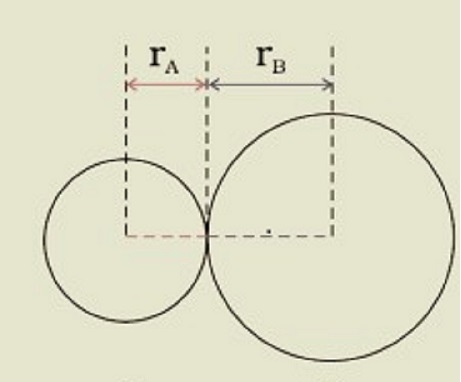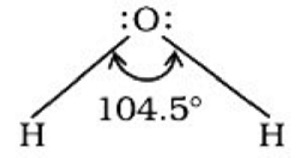# Bond Parameters

## Introduction

A variety of factors are used to evaluate covalent bonding. Bond length, bond strength, bond polarity, and bond multiplicity are only a few of them. Let's have a look at what these characteristics signify and how they affect us. Let's start with the bond length.

## Bond Length

The equilibrium distance between the nuclei of two bound atoms in a molecule is defined as bond length. The bond length is determined by each atom in the bonded pair. Each atom of the bonded pair participates in the bond length of a covalent bond. The contribution of each atom is referred to as the atom's covalent radius. In a bonded condition, the covalent radius is calculated as the radius of an atom's core in contact with the core of a neighbouring atom.$\mathrm{R=r_A+r_B}$ is the formula for the bond length in a covalent molecule AB, where r_A and r_B are the covalent radii of two atoms and R is the bond length. The covalent radius is defined as half the distance between two comparable atoms in the same molecule linked by a covalent bond. In a non-bonded condition, the van der Waals radius indicates the entire size of the atom, which includes its valence shell.

Furthermore, in a solid, the van der Waals radius is half the distance between two identical atoms in separate molecules. Covalent radii are always bigger than van der Waals radii.

Factors influencing bond length include -

• Because the distance between the valence shell electrons and the nucleus increases with the addition of electrons, bond length expands with the size of the atom.

For example,

$$\mathrm{C-C\:\lt\:Si-Si\:\lt\:Ge-Ge}$$

• Bond length reduces as bond multiplicity increases.

$$\mathrm{O=O\:\lt\:O-O}$$

## Bond Angle

The angle between the orbitals having bonding electron pairs around the central atom in a complex or molecule ion is defined as the Bond Angle. Bond angle is measured in degrees/minutes/seconds and may be obtained experimentally using spectroscopic techniques. It helps us determine the shape of a molecule/complex ion by giving us a sense of the arrangement of orbitals around the central atom.

In water, for example, the H–O–H bond angle can be expressed as follows −## Bond Order

The number of bonds between the two atoms in a molecule determines the Bond Order in the Lewis theory of covalent bonds.

For example, let us consider H2 which has one shared pair of electrons, O2 having two shared pair of electrons and N2 having three shared pairs of electrons, the bond order would be 1, 2 and 3, respectively. The bond order is also three in CO wherein there are three shared electron pairs between C and O.

Bond orders are equal for isoelectronic molecules and ions; for example, F2 and $\mathrm{O_2^{2−}}$ have bond order 1. The bond order of N2, CO, and NO+ is 3.

Bond enthalpy increases as bond order increases, but bond length decreases. It is important for understanding the stabilities of compounds.

## Bond Enthalpy

It's the amount of energy needed to break one mole of a specific sort of bond between two atoms in a gaseous state. Bond enthalpy is measured in kJ mol–1. The enthalpy of the H–H bond in a hydrogen molecule, for example, is 435.8 kJ mol–1.

$$\mathrm{H_2(g)\:\rightarrow\:2H(g) \:\:\:\:\:\:\:\Delta H^{\circ}=435.8\:kJ\: mol^{-1}}$$

$$\mathrm{N_2(g)\:\rightarrow\:2N(g)\:\:\:\:\:\:\:\Delta H^{\circ}=498\:kJ\:mol^{−1}}$$

$$\mathrm{O_2(g)\:\rightarrow\:2O(g)\:\:\:\:\:\:\:\Delta H^{\circ}=946\:kJ\:mol^{−1}}$$

The two factors affecting bond enthalpy are summarized below −

### Bond Length

The greater the value of bond enthalpy, the shorter the bond length. The length of a C-C bond is 154 pm, which is longer than the length of a C=C bond, which is 134 pm. As a result, the bond dissociation enthalpy of the C-C bond is 433 kJ/mol, whereas the bond dissociation enthalpy of the C=C bond is 619 kJ/mol.

### Atomic Size

The stronger the bond is, the smaller the bound atoms are. As a result, the bond dissociation enthalpy has to have a higher value. The bond dissociation enthalpy of the H-H bond, for example, is 435.8 kJ/mol, which is higher than that of the Cl-Cl bond, which is 243.5 kJ/mol.

The greater the bond dissociation enthalpy, the stronger the bond in the molecule will be. We have a heteronuclear diatomic molecule like HCl.

$$\mathrm{HCl(g)\:\rightarrow\:H(g) + Cl(g) \:\:\:\:\:\:\Delta H^{\circ}=431.0\:kJ\:mol^{1}}$$

The determination of bond strength in polyatomic compounds is more difficult. The enthalpy required to break the two O – H bonds in the $\mathrm{H_2O}$ molecule, for example, is not the same. The difference in the $\mathrm{\Delta H^{\circ}}$ value indicates that the second O – H bond changes as the chemical environment changes.

## Conclusion

While a single covalent bond is created when two atoms share an electron pair, multiple bonds are formed when two or three electron pairs are shared. Some bound atoms have extra pairs of electrons not involved in bonding.

Lone pairs of electrons are what they're called. The arrangement of bound pairs and lone pairs surrounding each atom in a molecule is shown in a Lewis dot structure. Bond length, bond angle, bond enthalpy and bond order, are all important factors connected with chemical bonds that have a substantial impact on the properties of compounds.

## FAQs

Q1. Mention some of the bond parameters effecting chemical bond.

Ans. The bond parameters affecting chemical bond are

• Bond Length

• Bond Order

• Bond Angle

• Bond Enthalpy.

Q2. Why Multiple Bonds are Stronger than Single Bonds?

Ans. As the quantity of bonding electrons between two atoms increases, the length of the bond between them shortens, resulting in the development of multiple bonds. The greater electron density between the atoms causes a stronger interaction between them, resulting in a decrease in bond length. The stronger the interaction, the stronger the bond. As a result, multiple bonds are more powerful than single ones.

Q3. What do you mean by the term Bond Length?

Ans. Bond length or bond distance is the distance between the centres of covalently bound atoms. X-ray diffraction of materials, electron diffraction, and spectroscopic (analysis of light absorbed/emitted by molecules) techniques are used to estimate bond lengths.

Q4. What is Van Der Waals Radii of Atoms?

Ans. In a non-bonded condition, the van der Waals radius indicates the entire size of the atom, which comprises its valence shell. Furthermore, in a solid, the van der Waals radius is half the distance between two identical atoms in separate molecules. Covalent radii are always smaller than van der Waals radii.

Q5. What is the trend followed by Single Covalent Radii in a Periodic Table?

Ans. It has been observed that single covalent radii increase down the group but decreases left to right along the period.

Updated on: 26-Apr-2023

162 Views